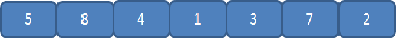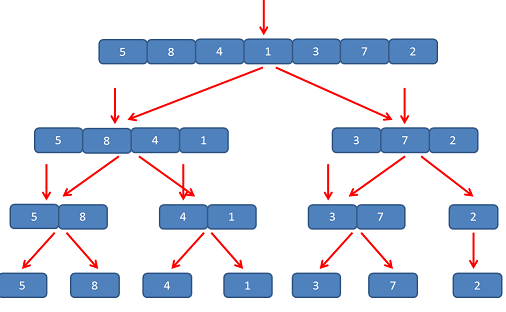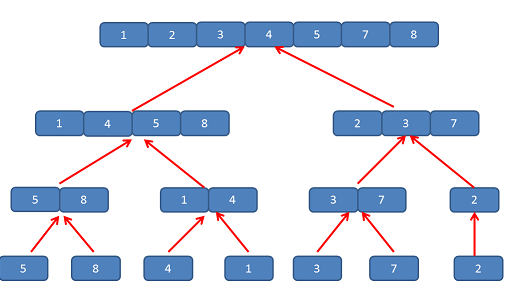# Merge Sort in Data Structure

This article explains Merge Sorting. So Let us understand the concept of Merge sorting, It’s Algorithm. How it works with an easy example.

## Points of Interest

• It is good at sorting large list of data
• It follows Divide and Conquer approach.

## Performance of Merge Sorting

It’s Best / Worst and Average Case Performance is same i.e., O(n log n)

## How Merge Sort Works

In this sorting technique, we divide the list in two equal (if list is even) or nearly equal (if list is odd) halves, recursively till it contains exactly one element left. And then compare each element with the adjacent list to sort and then merge the two adjacent list. And hence in this way finally we come up with the sorted list.

So let’s take an example and see step by step how it is working .

## Step by Step Working with Example

Let us take an array of 7 elements.Step 1 : Divide the list in 2 equal or nearly equal halves recursively till it contains one element in each sublist.Note: Here we are calculating the ‘mid’ (it is index from where we are going to split/ divide our list). mid = (lowest index + higher index) / 2 or for simplicity mid = (low + high)/2

Step 3 : Now compare each element with the adjacent sub-list one by one and start merging them to form a sorted list, starting from bottom.And that’s how you finally got array which is sorted and this algorithm does not sort in-place . means it requires extra memory to sort. And now you can copy all elements of new array in your original array.

Let us take a look on its algorithm

## Algorithm of Merge Sort

```Merge_sort(low, high) // low is starting index, high is end index mid = (low + high) /2 Merge_sort(low, mid) Merge_sort(mid+1, high)```

```/*Merge the sorted sublists*/ i = low j = mid + 1 k = low```

```Repeat until i>mid or j>high if A[ i ] < A[ j ] B[ k ] = A[ i ] // B is the new array increment i by 1 else B[ k ] = A[ j ] increment j by 1```

`increment k by 1`

```/*Find which sublist is exhausted and then copy rest elements of other list*/ if j > high , repeat 2 steps until i become greater than mid //means 2nd sublist gets empty B[ k ] = A [ i ] increment i and k by 1```

```if i > mid, repeat 2 steps until j become greater than high // means 1st sublist gets empty B[ k ] = A[ j ] increment j and k by 1```

So here is a video for additional understanding, if you still have some doubt in Merge sorting . or you can click on this youtube link : Merge Sort Video